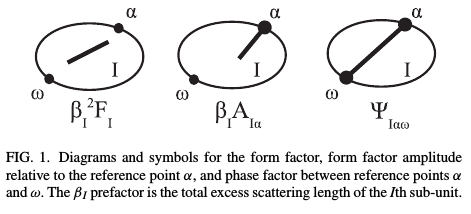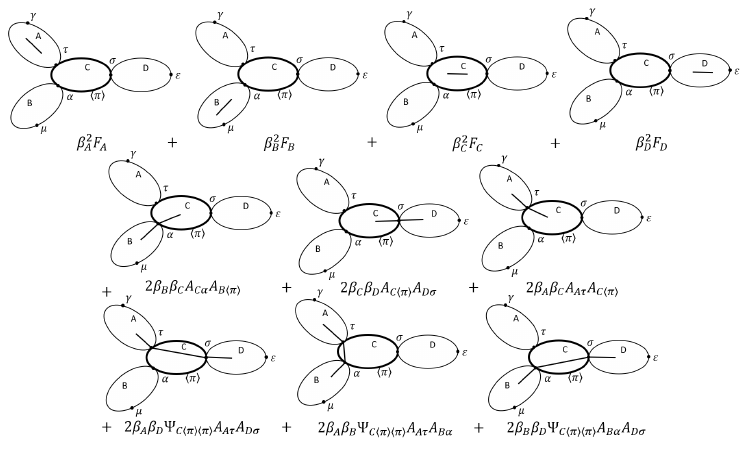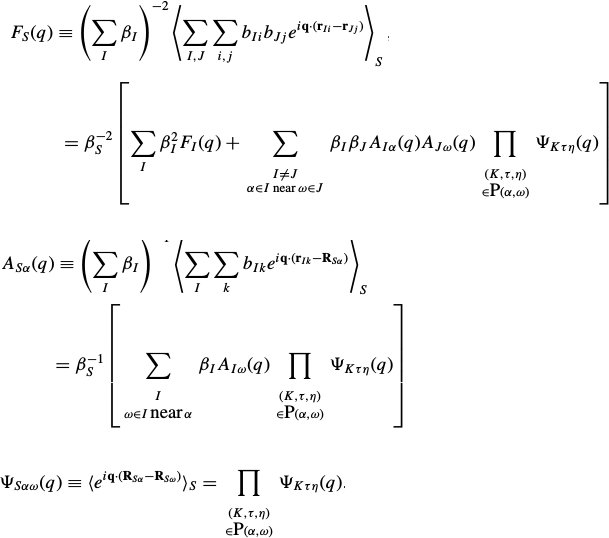## Scattering theory

Technique: Small angle Neutron, X-ray and light scattering are ideal techniques for characterizing the structure of colloids, polymers, and other self-assembled structures in solution. These techniques does not provide easy-to-interpret pictures of the structures, but rather difficult-to-interpret orientationally averaged scattering spectra. To analyze spectra extensive modelling is required by which the experimental results are compared to numerous theoretical models based on some assumed geometry of the structures present in the solution to determine the most likely structure.

Theory: The scattering spectrum depends on two functions. The structure factor encode how structures interact and it depends strongly on density. The form factor encode the 3D form or shape of a single structure. In a dilute solution, the scattering spectrum is entirely given by the form factor, and hence allows us to infer the structure from the scattering spectrum.

Liquid state theories such as PRISM allows structure factors to be predicted from complex structures, but require as input interaction potentials between sites in the structures as well as their form factor. pyPRISM is an example of a python code that can numerically solve the PRISM equations, and it implements form factors for some structures.

Problem: Our focus is on deriving expressions for form factors of structures modeled by connecting simple geometric sub-units together to form more complex structures. Deriving equations for the form factors of a different complex structures is mathematically a very complex and tedious process, and requires very friendly relations with a large table of Fourier transforms as well as numerous distributions and special functions. Structure factors then be predicted using PRISM formalism or approximating by estimating the overlap energy of radial distribution functions, that can also be predicted by our code.

Impact: Easy access to develop complex structural models which allows for more accurate, faster, cheaper analysis of experimental data, hence increasing the value of the experimental techniques.

Solution: Scattering Equation Builder is C++ library that Tobias Jarrett has implemented as part of his Bachelor thesis at SDU. It is based on a formalism described in the following papers

• "A formalism for scattering of complex composite structures. I. Applications to branched structures of asymmetric sub-units" Journal of Chemical Physics 136, 104105 (2012). Authors:  Carsten Svaneborg and Jan Skov Pedersen
• "A formalism for scattering of complex composite structures. II. Distributed reference points" Journal of Chemical Physics 136, 154907 (2012). Authors: Carsten Svaneborg and Jan Skov Pedersen

What does SEB do? We regard a structure as being modeled by geometric sub-units such as string like polymers, rods, and loops, or solid geometric objects such as disks, spheres, ellipses, cylinders and so on. These sub-units are connected at specific geometric points (joints). For instance polymers could be connected end-to-end to form a diblock copolymers, bottle brushes, stars or dendrimer structures. Almost any branched structure of block-copolymers can be build in this way. By attaching polymers to the surface of geometric objects as spheres or cylinders, we can build block-copolymer micelles or worm-like micelles. To derive the form factor of any of these structures analytically is hard work. With SEB it is just a few lines of code to define the structure, and the output is an analytic expression for the form factor. Such expressions can be used for fitting experimental data.

What does SEB NOT do?  SEB is a library, it is not a program with a graphical user interface written for users. SEB can not read data nor perform parameter fitting. Other libraries can supply these generic functionalities. Our focus is to supply the main component for the analysis of scattering data analytic form factor models flexibly and efficiently.

How does SEB work? The library keeps tracks of all the details of which types of sub-units are present in a structure. All sub-units have a specific type, and a unique name. All joints in a sub-unit also have unique name. Currently the user can write a C++ program and grow a structure by repeatedly adding sub-units to the structure by connecting a joint on each new sub-unit to an existing joint in the structure. Structures can contain an arbitrary number of sub-units in an arbitrary connected tree structure (as long as it is acyclic, see Caveats below).

All mathematical expressions are represented via GiNaC expressions, which means all mathematical calculations are made exactly and analytically as in any CAS tool. Hence the form factors produced by SEB are analytic mathematical expressions which can e.g. involve integrals for explicit orientational averages and special functions. Hence to derive the radius of gyration of a structure, we can utilize GiNaC to Taylor expand the form factor and hence we can also produce an analytic expression for the radius of gyration.

The user can also specify structural parameters, e.g. the radii of gyration for polymers, length of rods, length and radius of cylinders etc. and when these are inserted into a generic form factor expression F(q; structural parameters), the result is an analytic expression F(q) which depends on a single parameter. Finally specifying the q value, the form factor can be evaluated to a number, even if the expression involves integrals.

Hence the user can with very few lines of code generate almost any structure, e.g. any of the structures above. When the structure has been defined, the user can ask for

1. The value of the form factor of the structure evaluated for specified set structural parameters evaluated for a given momentum transfer q value.
2. The equation of the form factor in LaTeX format with several levels of abstraction e.g. as a single structural equation and separate scattering functions for each sub-unit, or as a single very large equation.
3. A pointer to a C++ function that evaluates the form factor that can be used e.g. in a fit program.
4. A GiNaC expression that can be used for additional CAS transformations.

Besides the form factor, the user can also get all of the above for the form factor amplitude and phase factors for the whole structure.

The form factor amplitudes can be used to estimate the radial (excess scattering length) density profile relative to any joint in the structure. Assuming a structure that has a meaningful geometric center, such as a star, a dendrimer or a micelle with a special core. Then the simplest guess for the structure factor would be S(q)=Ac2(q) Scc(q) where Ac would be the form factor amplitude relative to that center, and Scc(q) related to the Fourier transform of the potential of mean force between the centers.

The phase factors contains information about the average distance between pairs of joints. Hence they can be used e.g. to calculate the distribution of distances between the free ends of polymers attached to a spherical surface to model the structure of a block-copolymer. This could e.g. be relevant to predict the Fluorescence signal of a FRET experiment, if the free ends of the polymers were labelled with donor-acceptor pairs.

Modularity: The C++ library define a parent sub-unit class, that can be used for deriving concrete sub-units with very little additional code beyond the mathematical expressions required for their scattering functions. The formalism is also closed in the sense that any structure can be build by connecting sub-units can also be used as a sub-unit. E.g. if we build a star by connecting N rods to a common center, then the star can be used as a sub-unit to build a chain of M stars.

Near future: Currently, we have developed the core functionality and a few sub-units. We will be implementing many more sub-units, and also the option to join not only pairs of geometric points, but also join on lines, surfaces and volumes. E.g. join 100 short polymers by one end to a random point along the contour of a loop-polymer to create a loop bottle brush, or join them on the surface of a cylinder to create a work-like micelle. We would also like to implement generic containers like linear or star taking a specific sub-unit as an argument, which would simplify the code and shorten scattering equations.

More distant future: We would like to develop a programmatic interface, such that one does not to write and compile a C++ program for each structure, but can instead supply the library with a small "program" defining sub-units and their connections. We would also like to develop a python wrapper to make it easy to integrate SEB and pyPRISM. We would also like to implement a sub-unit representing a rigid cloud of scatteres, i.e. as a table of pair-distances and products of scattering lengths, such that we have a generic sub-unit for modelling e.g. proteins in solution. Being able to export equations to Mathematica or Matlab. Perhaps automatically generate Pov-Ray visualizations of the structure or export to WebGl such that examples of the structures can be visualized.

Under the hood: All scattering techniques measures distances between pairs of scatterers. For a structure composed of sub-units, we can decompose these distances into intra-subunit distances (described by the form factor of the sub-unit), and inter-subunit distances, which are described by the form factor amplitudes of the two sub-units in question, and the phase factors of all the sub-units linking the two together in the structure.

We can illustrate these contributions using diagrams quite similar to Feynmann diagrams known from high energy physics. For a single sub-unit (I ellipse below), the form factor, form factor amplitude (relative to joint alpha) and phase-factor (relative to alpha, and omega) of a sub-unit can be shown with diagrams such as those below. Beta's denote the total scattering length of the sub-unit I. For the form factor the line inside the sub-unit illustrate a pair difference between any pair of scatterers inside the sub-unit. Points on the "surface" of the sub-unit are potential joints (denoted reference points in the papers and code). A joint could be a geometric point such as one of the ends of a polymer chain, or it could be a random point on the surface or equator line of a sphere. Hence the line in the form factor amplitude illustrate the distance between any scatterer in the sub-unit and a specified joint (here alpha). The formalism require a mathematical expression for the form factor amplitude relative to each joint. Finally phase factors contain information about the distance between pairs of joints. This distance could be a constant e.g. for the end-to-end distance of a rod, but could also be characterized by a distribution e.g. the end-to-end distance of a polymer. The formalism requires mathematical expressions for phase factors between all unique pairs of reference points. For geometric reference points, the phase factor between a point and itself is unity, but if reference points are scattered randomly on a polymer chain, then the expression for the phase factor is identical to the form factor.Having established the diagrams for sub-units, we can connect these sub-units together by pairs of joints to form almost any complex structures of interest. Below is shown an example of all the scattering contributions from a structure composed of a central unit with three arms attached to it. Also illustrated are all the pair distances between scatterers diagramatically and the corresponding analytic expressions. (from the Bachelor thesis of Tobias Jarrett)With a bit of mathematics, its can be shown with certain assumptions (see below) the form factor of any structure composed of sub-units can be expressed in terms of the form factors, form factor amplitudes, and phase factors for those sub-units in fact the result is the general equation below. The illustration above shows a particular example of this equation.Caveat: Nothing is perfect.

To derive these equations, we had to assume

1. That all sub-units are mutually non-interacting.
2. All joints are completely flexible.
3. The structure is  acyclic.

The consequence of these assumptions are that the internal conformations of the sub-units are statistically uncorrelated which is required for the factorization of the contributions. If two sub-units interact, e.g. excluded volume interactions between two blocks in a di-block copolymer then there will be conformational correlations between the two blocks, in which case the factorization used above is not exact. It might still be the best available approximation, but e.g. extensive computer simulations would be required to investigate the quality of the approximation. If joints are not flexible, then there would obviously also be configurational correlations between two rigidly joined rods. Finally cyclic structures also contain conformational correlations since there is an additional closure constraint on the internal conformations.

Note that no assumptions are made wrt. the internal structure of a sub-unit. Hence if we knew the scattering functions of a polymer with excluded volume interactions, we could use excluded-volume polymer conformational statistics inside a sub-unit.